## Polychora

"As for the analogous figures in four or more dimensions, we can never fully comprehend them by direct observation. In attempting to do so, however, we seem to peep though a chink in the wall of our physical limitations, into a new world of dazzling beauty."
H. S. M. Coxeter, in the preface of "Regular Polytopes".

The generalization of "polygon" in 2 dimensions and "polyhedron" in 3 dimensions to any number of dimensions is a polytope. A 4-dimensional polytope is a polychoron (plural "polychora"). This is a finite region of 4-D Euclidean space* bound by at least 5 polyhedral 3-faces, or cells; it must also have at least 5 vertices, 10 edges and 10 faces. Edges link two vertices; likewise, cells meet in pairs along their common faces. Faces and cells meet in equal numbers and in sets of at least 3 each at each edge in a way specified by its polygonal figure (henceforth "edge figure"). Just as each polyhedral cell is limited by at least 4 faces, 6 edges and 4 vertices, at least 4 cells, 6 faces and 4 edges meet at each vertex in a way specified by a single polyhedral figure (henceforth "vertex polyhedron"). These surface elements are, together with the inner 4-D ``volume", part of the polychoron.

Regarding the vertex polyhedron: Its faces correspond to vertex figures of the cells of the polychoron that touch that vertex. Its edges correspond to faces of the polychoron that touch that vertex. Its vertices and vertex figues correspond to the edges and edge figures of the polychoron that meet at that vertex.

If two polychora A and B are duals, then to each vertex of A corresponds a cell in B, which is the dual of the vertex polyhedron of A, and vice-versa. This implies that the cell's vertex figure (i.e., the face of the vertex polyhedron) is the same for two dual polychora! Each edge of A goes through a perpendicular face of B, which is identical to that edge's figure, and vice-versa. Two dual polychora must necessarily have the same symmetry.

* This is, again, a traditional definition that excludes honeycombs, which are infinite divisions of a 3-D Euclidean space. However, polychora are closely associated with tesselations of the 3-sphere, which are also finite.

### Projections

The models below are orthographic projections of polychora to 3-dimensional space that can be built with the Zometool system, i.e., that are "Zomable" (if a polychoron has a Zomable projection, we will likewise say it is Zomable). The projections shown below are done along a vector that is perpendicular to our own 3-D ``plane''.

In these projections, most or all elements are necessarily distorted, in the same way that the projection of a Cube to a flat surface will necessarily distort most or all of the faces and edges of the Cube. A way of seeing which elements are not distorted is by their colour: green struts in the models containing them, blue in the models without (i.e., the colours of the longer struts in the model). This only applies, of course, to cases where all edges of the polychoron being projected have the same length, which is true for all polytopes in this site.

Such undistorted elements thus lie in 3-D ``planes'' parallel to our own. If undistorted cells occur, they invariably appear at the center of the projection; they are the ``closest'' and ``farthest" away from our 3-D ``plane'', and the projection is ``cell-first''. Below, we also show ``vertex-first'' projections (with a connector at the center), which show undistorted vertex polyhedra. Occasionally we show other types of projections.

If larger undistorted polyhedra occur in the projection, they represent cases where specific sections of the polychoron (by additional 3-D planes that are parallel to ours) happen to be highlighted by its edges. An equivalent of this in 3-D would be the flat Pentagons we can see surrounding each vertex in the Icosahedron. If one of these envelops the whole projection, then it is an ``equatorial'' polyhedron, the section by a 3-D ``plane'' that is halfway between two opposed central elements. An equivalent of this in 3-D are the equatorial Polygons of the rectified polyhedra.

All regular polychora have Zomable projections, below we show all of them. In addition, we show a few Zomable projections of some closely related forms. Since all these projections have a lot of important internal structure, there is no alternative to building them in Zometool: a paper model would hide the internal structure of the projection. We will refer to the specific projections by their 3-D symmetry: thus, a projection with Tetrahedral symmetry will be described as a Tetrahedral projection; and likewise for all others.

### Regular and uniform convex polychora

In a regular polychoron all cells and vertex polyhedra are regular. This implies that it is isochoral: the cells are not only identical, but the polychoron looks the same seen from all of them; this implies, for instance, that all cells are equidistant from its centre. The same is true for the other types of elements, i.e., the polychoron is also isogonal, isotoxal and isohedral.

There are sixteen regular polychora: six are convex and ten are non-convex. Some have many names - one is known as the 120-cell, Dodecaplex, Hyperdodecahedron, Polydodecahedron, Hecatonicosachoron, Dodecacontachoron and Hecatonicosahedroid. The convention adopted here is the same adopted by Wikipedia, which is to use the simpler names, for this example "120-cell". This is consistent with the names of the regular polyhedra, which refer only to the number of faces, not their type. This simple system works because the resulting names are unambiguous. Furthermore, "120-cell" is much easier to understand, and shorter to write, than the equivalent Greek name, "Hecatonicosachoron". The only exception is that for the 8-cell we will use the widely known term "Tesseract". This is also consistent with the polyhedral and polygonal names, where the terms "Cube" and "Square" are at the same time technically precise (they imply regularity) and do not reflect the number of faces or edges.

Apart from the regular polychora, we will also show a few of their rectifications (but not of their duals). These are uniform polychora: they are isogonal and all cells are uniform polyhedra, which are also isogonal. But apart from that, these rectifications are also isotoxal, which implies that all vertex polyhedra, apart from being identical, are also isogonal. The number of their vertices is the same as the number of edges of the regular polytope that was rectified. One of the differences is that, while for each pair of dual regular polyhedra there is one rectification, for two dual regular polychora (or any higher dimensional polytope) there are two distinct rectifications.

***

The 5-cell is the 4-D analogue of the Tetrahedron. It is bound by 5 Tetrahedral cells and has 5 vertices, with Tetrahedral vertex polyhedra. These cells have 5 × 4 = 20 Triangular faces, however, each face is shared by two cells, so the total numbers of faces is 10. The cells also have 5 × 6 = 30 edges, however, the edges have Triangular figures, meaning that each edge is shared by three cells, resulting in a total of 10 edges. The symmetry of the numbers of elements (5-10-10-5) and the duality of the Tetrahedral cells and Tetrahedral vertex polyhedra imply that the 5-cell is, like the Tetrahedron, self-dual. Its Tetrahedral projection is shown in Fig. 15.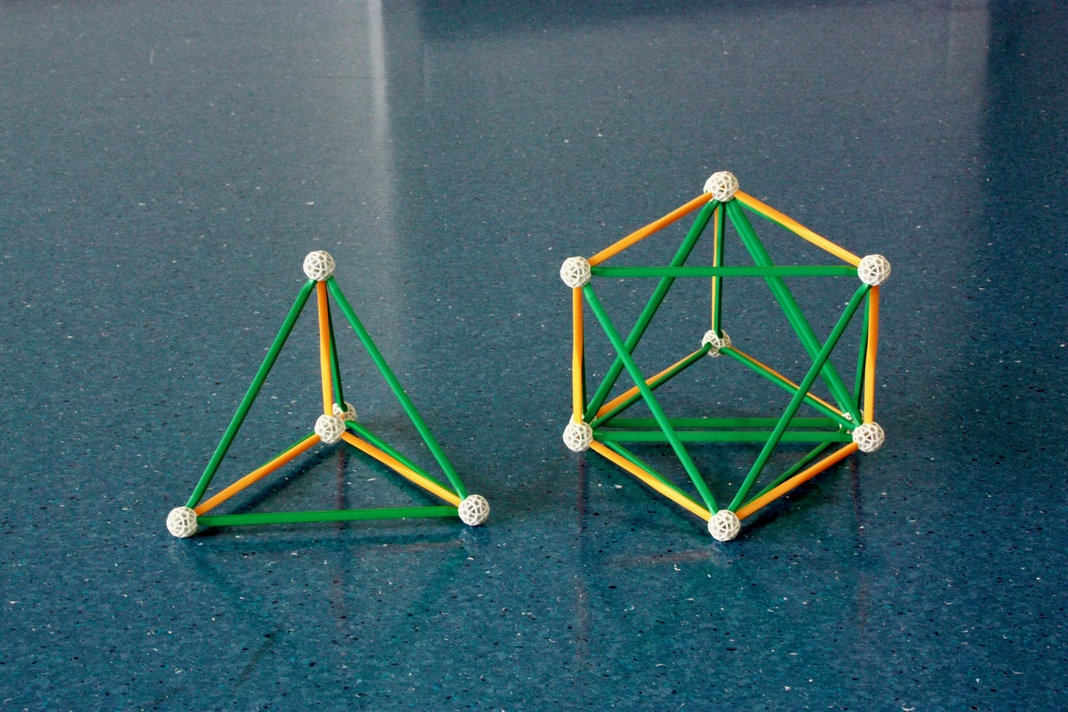Fig. 15: Projections of the 5-cell and its rectification.

Left: A "vertex-first" and "cell-first" Tetrahedral projection of simplest regular polychoron, the 5-cell. Right: A Tetrahedral (thus, cell-centered) projection of the Rectified 5-cell.

For each of the 5 Tetrahedra of the 5-cell, the Rectified 5-cell has 5 rectifications of the Tetrahedron, or Octahedra. Each vertex of the 5-cell is replaced by its vertex polyhedron, a Tetrahedron, two of each touch the new vertices from opposite directions. The number of these new vertices is the same as the number of edges of the 5-cell, 10; their vertex figure is the Triangular prism because of the Triangular vertex figures of the new Tetrahedra, the Square ``sides'' are the vertex figures of the new Octahedra. Since all its cells are regular, the Rectified 5-cell is one of the three Semi-regular convex polychora. Together with the Triangular prism, it is a member of the finite multi-dimensional k21 family of Semi-regular polytopes.

Both polytopes in Fig. 15 have Pentachoric symmetry. For more Zomable projections of the 5-cell, see Fig. 35.

***

The 16-cell, the 4-D analogue of the Octahedron, is bound by 16 Tetrahedral cells and has 8 vertices with Octahedral vertex polyhedra. Following the logic used above to calculate the number of elements of the 5-cell, we find it has 24 edges with Square figures, and 32 Triangular faces. Its dual, the Tesseract, is the 4-D analogue of the Cube. This is bound by 8 Cubic cells and has 16 vertices with Tetrahedra as vertex polyhedra. It has 32 edges with Triangular vertex figures and 24 Square faces.

In Figs. 16a and b, we show in the front rows different Octahedral projections of the 16-cell and Tesseract. In the back rows we show projections of their rectifications, specifically the rectifications of these specific projections. For more Zomable projections of the 16-cell, see Figs. 28a and b. For more projections of the Tesseract, see Fig. 30.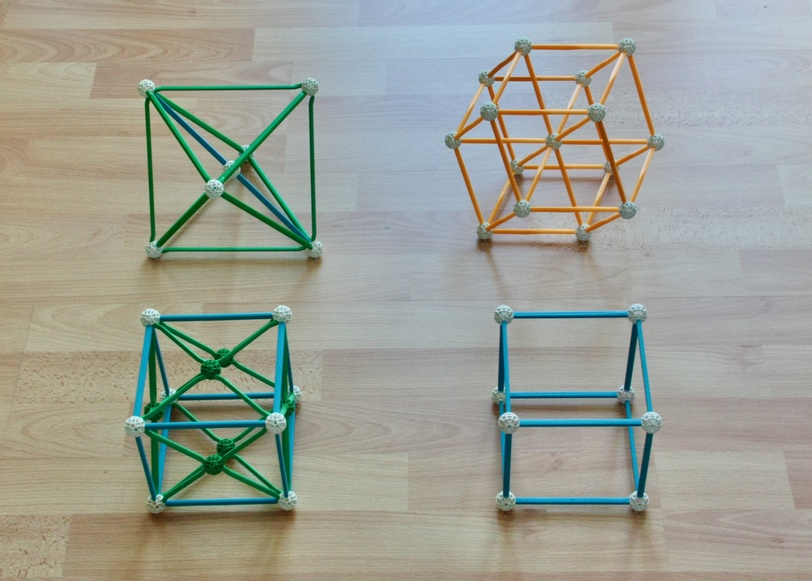Fig. 16a: Models of vertex-first projections of the 16-cell, Tesseract and their rectifications.

Front Left: The 16-cell. Note the envelope, which represents the equatorial polyhedron, the Octahedron. Three flattened projections of the Octahedron also appear in the three perpendicular planes passing through the centre.
Front Right: The Tesseract.

Back Right: The Rectified tesseract. Each of the 8 Cubes of the Tesseract is replaced with rectifications of the Cube, Cuboctahedra. Each of the 16 vertices of the Tesseract is replaced by the Tesseract's vertex polyhedron, the Tetrahedron. Two of these Tetrahedra appear in the centre of the projection.Fig. 16b: Cell-first projections of the 16-cell, Tesseract and their rectifications.

Front, left: The 16-cell. This projection is especially good for highlighting its 8 vertices separately.
Front right: The Tesseract. In this projection, two Cubes, one "near" and the other "far" from our own 3-D ``Plane", appear perfectly superposed and without distortion. The other six Cubes are flattened into the six Square faces. This can be understood very well by imagining the shadow of a Cube in a 2-D surface, where it can be made to resemble a Square, bordered by flattened faces.

Back right: Rectification of the cell-centered projection of the Tesseract. Notice the six flat projections of Cuboctahedra at the surface of the model.
Back left: Rectification of the cell-first projection of the 16-cell.

Note: the two projections of the Rectified tesseract in this and the previous Figure have been disassembled.

We described above the meaning of duality between polychora. We now describe the meaning of duality between projections. The cell-first projection of the Tesseract in Fig. 16b is the dual of the vertex-first projection of the 16-cell in Fig. 16a; the reason is that each cell of the former is centered exactly on a vertex of the latter, and vice-versa. Equally, the cell-first projection of the 16-cell in Fig. 16b is the dual of the vertex-first projection of the Tesseract in Fig. 16a. Two dual projections necessarily have the same symmetry.

These regular polychora have analogues not only in 3-D, but also in any dimensional space. The analogues of the 5-cell are known as Simplexes, all of them self-dual. The Tesseract is the 4-D Hypercube, and the 16-cell is a 4-D Orthoplex. These polytope families are infinite. Among these, the 16-cell is especially interesting: its vertices represent half of the vertices of the Tesseract, making it, together with the Tetrahedron, a member of yet another infinite family of polytopes, the Demi-hypercubes. Also, as one might expect, Tesseracts can fill 4-D space, but the 16-cell can do that as well: it is the only member of this infinite family that can fill the Euclidean space where it exists! The vertex arrangement of this honeycomb is known as the F4 lattice. If a 3-sphere is centered on the vertices of this lattice, the resulting arrangement is the densest possible regular sphere packing in 4 dimensions, with kissing number 24.

The four polytopes in Figs. 16a and b share the Hexadecachoric symmetry, but one of them (the Rectified 16-cell) has a higher degree of symmetry. That this object is special can be seen in a detail in Figs. 16a and b: while the two projections of the Rectified tesseract are different (the first is centered on a Tetrahedron, the second is centered on a Cuboctahedron) the projections of the Rectified 16-cell in both Figures are identical. We now discuss this object in more detail.

***

Ultimately, the Rectified 5-cell owes its semi-regular status to a fact we mentioned when discussing polyhedra: the rectification of a Tetrahedron is another regular solid, the Octahedron. This means that, when we rectify any regular polychoron with Tetrahedral cells, we obtain a semi-regular polychoron bound by Octahedral cells and cells identical to the former's (regular) vertex polyhedron. As seen above for the Rectified 5-cell, the vertex polyhedra are equilateral prisms, with a "base" given by the edge figure of the regular polychoron that was rectified.

Things are even more interesting when we rectify the 16-cell. Doing this, we create a polychoron where 16 Octahedral cells result from rectifying the Tetrahedra of the 16-cell (the projection in Fig. 16a is centered on these) and where 8 new Octahedral cells are the vertex polyhedra of the 16-cell (the projection in Fig. 16b is centered on these). The vertex polyhedron is the Square prism, i.e., the Cube. This means that this polytope is regular: the 24-cell (see Fig. 17). This is the reason why the projections in Figs. 16a and b are identical. There is an even deeper, and rather beautiful reason for this, triality, which is a result of its Icositetrachoric symmetry.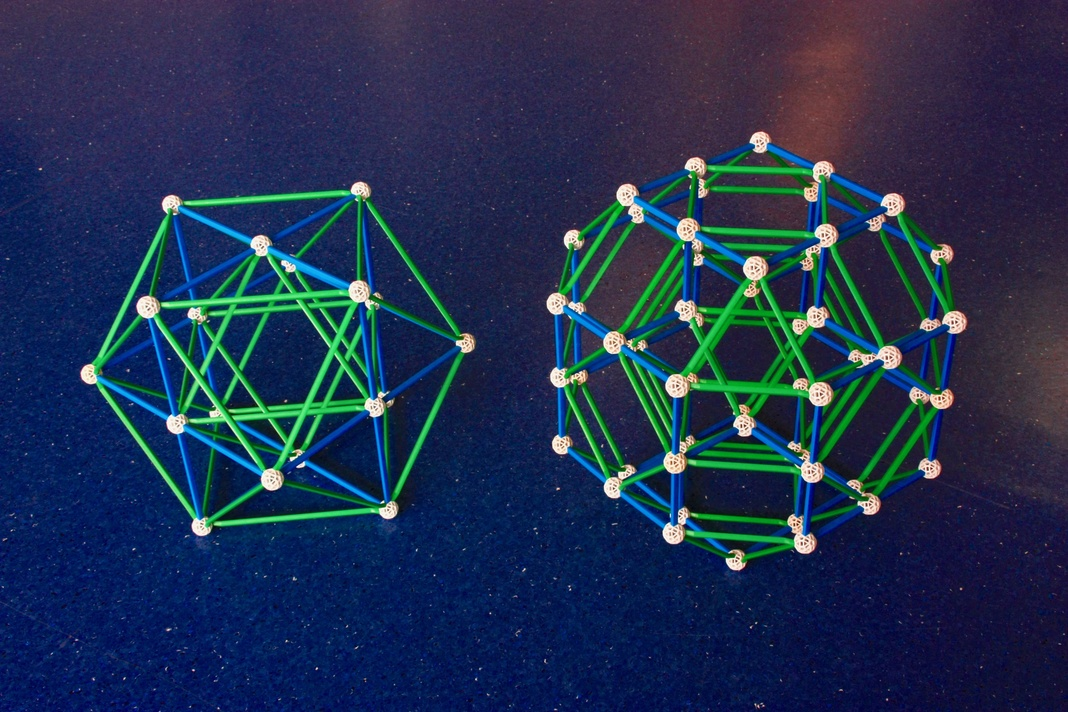Fig. 17: Left: Yet again, an Octahedral projection of the Rectified 16-cell, more commonly known as the 24-cell. Note the equatorial polyhedron, the Cuboctahedron. Note too the three other flat projections of Cuboctahedra in three orthogonal planes passing through the centre.

Right: An Octahedral cuboctahedral-cell-first projection of the Rectified 24-cell.

How to build: Have a look at the Eusebeia page on the Rectified 24-cell. For this projection, see the part on the "Cantellated 16-cell", for the pyritohedric projection, see the first part of the page. This model has been disassembled.

Because it is a rectification of the 16-cell, the number of its vertices has to be the same as the number of edges of the 16-cell, also 24. The number of edges (which have Triangular figures) and Triangular faces is 96. All of this implies that the 24-cell is self-dual. In all Euclidean n-dimensional spaces, this is the only regular convex polytope with this characteristic that is not a Simplex. Furthermore, the 24-cell and its symmetry have no analogs in any other n-dimensional space, i.e., the 24-cell and its symmetry are clearly exceptional objects! We will now find why.

The previous way of building the 24-cell (by rectifying the 16-cell) is known as Cesàro's construction; this is the 4-D equivalent of obtaining a Cuboctahedron from an Octahedron by rectification. The "dual" construction of the 24-cell (Gosset's construction) is equivalent to the construction of a Rhombic dodecahedron from two Cubes mentioned above. This construction illustrates especially well why the 24-cell is unique to 4 dimensions.

The first step is the division of a Tesseract into 8 Cubic pyramids. Their 4-D volume is limited by a Cubic "base" (one of the previous cells of the Tesseract) and by 6 Square pyramids, the "sides", the apex lies 1/2 edge length above the base. In a second step, we separate these Cubic pyramids and "glue" their Cubic bases to the Cubic cells of a second Tesseract, making those - and all Square faces - disappear. Square pyramids from two neighbouring Cubic pyramids now contact each other through what were their Square bases, thus forming 24 Octahedra - the cells of the 24-cell. The apices of these pyramids are 1 edge length from the center of the former Tesseract.

As shown by the construction of the Rhombic dodecahedron from Cubes, similar constructions can be made in any dimensional space. The difference is that among hypercubes in all n-dimensional spaces, the Tesseract is the only one where the distance from the vertex to the centre (given by √ n / 2 edge lengths) is 1 edge length, i.e., it is radially equilateral. This means that the Cubic pyramids and their Square pyramid "sides" used in the previous steps are equilateral, a necessary pre-condition for building Octahedra.

To the 16 vertices of the original Tesseract, all 1 edge length from the center, 8 new ones were added: the apices of the Cubic pyramids, which lie "above" each Cubic cell of the original Tesseract also 1 edge length from the center. Thus, the 24-cell is, like the Tesseract, radially equilateral.

These 8 apices have the vertex arrangement of the dual of the Tesseract, the 16-cell. Thus, the 24-cell has the vertices of a dual compound, the Tesseract and the 16-cell. Furthermore, as we've seen above, we can divide the vertices of the previous Tesseract into those of another two 16-cells, therefore the vertices of the 24-cell can be decomposed into three sets of vertices of the 16-cell. As we will see, a set of 3 16-cells with the vertices of the 24-cell is a regular polychoron compound (see Figs. 29a, b and c).

Note that, although all Square faces disappeared in the last stage of Gosset's construction, their vertices and edges are still present in the 24-cell as the Square ``equators" of the Octahedra. Since each Octahedron has three such equatorial Squares, the edges and vertices of the 24-cell are the edges and vertices of three different Tesseracts, which together make another regular polychoron compound, the dual of the aforementioned Compound of three 16-cells (see Fig. 30).

As suggested by their derivations, the Cuboctahedron and Rhombic dodecahedron are the closest polyhedral analogues to the 24-cell. The Cuboctahedron is the envelope of the cell-first projection of the 24-cell in Fig. 17 because it is the application of Cesàro's construction (rectification) to the Octahedral envelope of the projection of the 16-cell in Fig. 16a. Being represented by the green struts means that it is undistorted and that its 12 vertices (half of the vertices of the 24-cell), edges and faces are in the same 3-D ``plane'' parallel to ours; being an envelope of the projection means that it is the ``Equatorial'' polyhedron of the 24-cell, which means that its 3-D plane contains the centre of the 24-cell. The lines going from this centre to the vertices of this Cuboctahedron thus appear, like its edges, undistorted; this implies that the Cuboctahedron must be, like the 24-cell, radially equilateral (see Fig. 27). The Cuboctahedron also has an equatorial polygon, the Hexagon, also with 1/2 of its vertices, which is also radially equilateral. Apart from the Tesseract and 24-cell, the Hexagon is the only regular polytope with that property.

The Rhombic dodecahedron is the envelope of the vertex-first projection of the 24-cell (see Fig. 29b) because it is the application of the Gosset construction to the Cubic envelope of the Tesseract in Fig. 16b. Like the Rhombic dodecahedron, the 24-cell can fill space. To see this, we apply the same process used above to create the Rhombic dodecahedron honeycomb from the Cubic honeycomb to a Tesseractic honeycomb: First, we divide half the Tesseracts of the honeycomb (which touch each other only at their vertices) into 8 Cubic pyramids. Second, we attach their Cubic bases to the Cubic cells of its 8 neighbouring Tesseracts, thus making 24-cells via Gosset's construction above. This is the regular Icositetrachoric honeycomb. Its dual must also be regular; it is in fact the 16-cell honeycomb mentioned above. Thus, it becomes clear why 4-D space is the only Euclidean space in any dimensions with three regular honeycombs; all others have only their respective Hypercubic honeycomb!

***

As we've seen, the 24-cell is unique to 4 dimensions. As we will see now, its existence is a necessary condition for the existence of the more complex regular polychora, being thus fundamental for understanding the exceptional richness of the fourth dimension. The construction of the more complex polytopes from the 24-cell is known as Gosset's construction, which we now describe (see Coxeter 1973).

Step 1: Rectify the 24-cell, obtaining the Rectified 24-cell (Fig. 17). For each of the 24 Octahedra of the 24-cell the latter has one Cuboctahedron (the rectification of the Octahedron). For each of the 24 vertices of the 24-cell, the Rectified 24-cell has a Cube (the vertex polyhedron of the 24-cell). This polychoron has 96 vertices, one for each edge of the 24-cell.

Step 2: Diagonally bisect all of its Squares. If the diagonals are chosen correctly, then each of the 12 vertices of the Cuboctahedral cells has 5 edges and five triangular faces converging on it, i.e., it has been transformed into an irregular icosahedron (see Fig. 18). The Cubic cells of the Rectified 24-cell have been transformed into sets of 5 Tetrahedra, with only the central one being regular.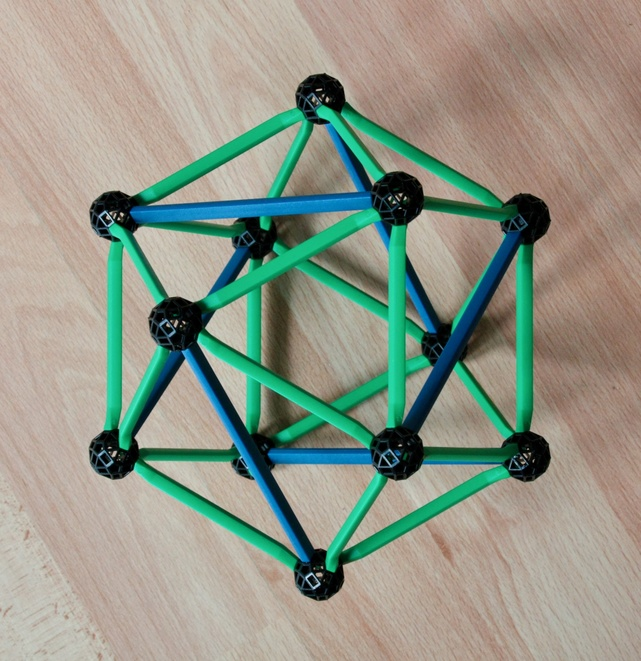Fig. 18: Being a rectification of the Octahedron or Cube, the Cuboctahedron (in green, see also Fig. 4) has 12 vertices, the number of edges of the Cube and Octahedron. This is the same number of vertices as the Icosahedron. That is why their duals are both dodecahedra, respectively the Rhombic dodecahedron (Fig. 4) and the Dodecahedron (Fig. 2).

The reason for the identical number of vertices is that if we add new edges along the diagonals of the Square faces of the Cuboctahedron as shown here (in blue), it becomes an irregular Icosahedron, with 5 edges and 5 triangles converging on each of its vertices. By shortening those diagonals to the size of the other edges, we transform the irregular icosahedron into a regular one. None of these operations changed the number of vertices.

Step 3: Shorten these Square diagonals until they have the same length as the other edges. This generates one regular Icosahedron for each of the previous 24 irregular icosahedra, and the 24 clusters of mostly irregular tetrahedra become clusters of 5 (regular) Tetrahedra, 120 in total. The resultant polychoron is the Snub 24-cell (Fig. 19). The second and third operations preserved the number of vertices of the Rectified 24-cell, 96, all of them are still identical, the vertex polyhedron is a Johnson solid, the tri-diminished icosahedron. This means it is still a Uniform polychoron. Because all cells are regular, it is also semi-regular. However, and unlike the polychora represented by the models above, this is neither regular nor derived from a regular polychoron by rectification. Its symmetry is no longer the symmetry of the 24-cell, it is instead a ionic diminished icositetrachoric symmetry.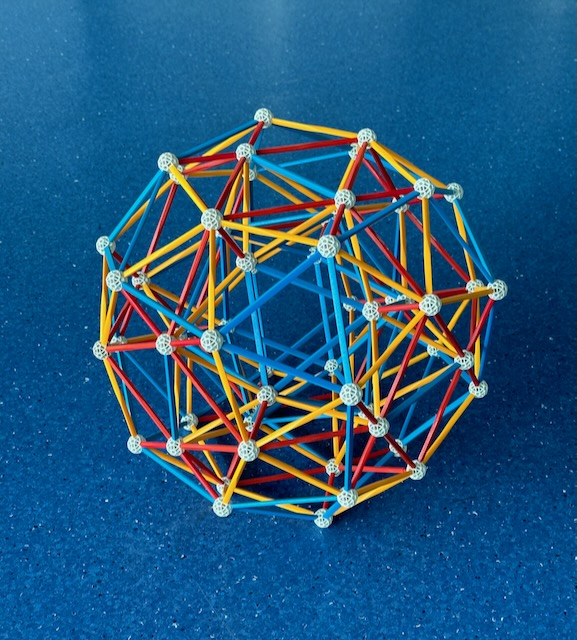Fig. 19: This is an icosahedral-cell-first projection of a convex uniform polychoron, the Snub 24-cell.
Picture by Jason Wu.

How to build: Have a look at the Eusebeia page on the Snub 24-cell.
Also recommended is David Richter's page on the 24-cell and its snub.

Now, on the symmetry of the model in Fig. 19. When we choose the diagonals of the Cuboctahedron, we break its Octahedral symmetry. In this case, we have only 3-fold rotational symmetry around the triangular faces (not full reflection symmetry), and for each Square face, we have now a rectangular symmetry, not a full Square symmetry. This is, again, the pyritohedral symmetry. This remains as the overall symmetry of the model of the Snub 24-cell in Fig. 19 even after the transformation of the irregular icosahedra into Icosahedra.

Step 4: Glue a Icosahedral pyramid (consisting of an Icosahedral "base" and 20 regular Tetrahedra as "sides", see Fig. 20) by its base to each of the 24 Icosahedral cells of the Snub 24-cell. The Icosahedral cells therefore disappear.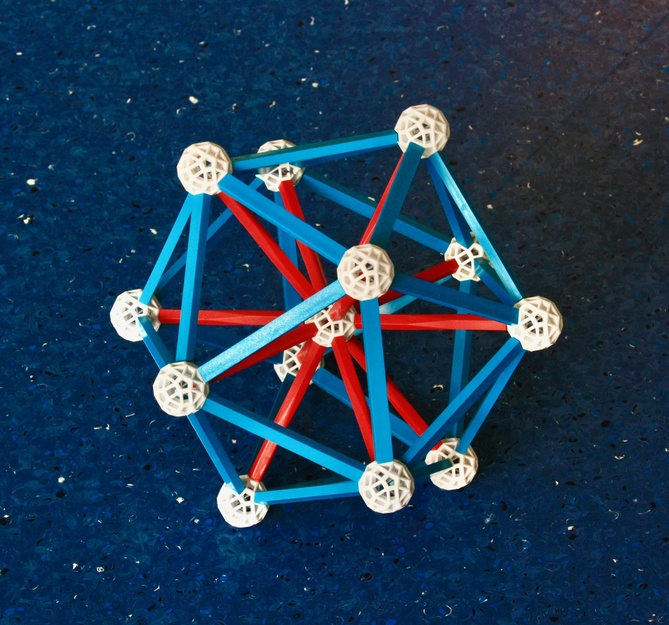Fig. 20: Icosahedral projection of the Icosahedral pyramid. This can be seen as an "apex-first" projection, but also as a "base-first" projection, where the base is the outer Icosahedron. This pyramid can be equilateral (in 4-D) because the Icosahedron's circumradius (red struts) is slightly smaller than its edge length (blue struts).

The 120 Tetrahedra of the Snub 24-cell are now joined by the 24 × 20 Tetrahedra = 480 Tetrahedra of the new 4-D "Pyramids", making a total of 600 Tetrahedral cells. Together with the previous 96 vertices of the Snub 24-cell, the new 24 pyramidal apices add to a total of 120 vertices. The former's vertex polyhedra are still identical; in fact they have all become regular: 20 Tetrahedra now meet at each vertex, which means that the vertex polyhedron is an Icosahedron. This is also the case for the 24 new pyramidal apices!

Since all cells and vertex polyhedra are regular, we have built a new regular polychoron, the 600-cell! It has 1200 Triangular faces and 720 edges with Pentagonal figures. This is the 4-D analogue of the Icosahedron; its symmetry, known as the Hexachosichoric symmetry, is the 4-D analogue of the Icosahedral symmetry. Its Icosahedral projection is shown in Fig. 21; this is necessarily a "vertex-first" projection.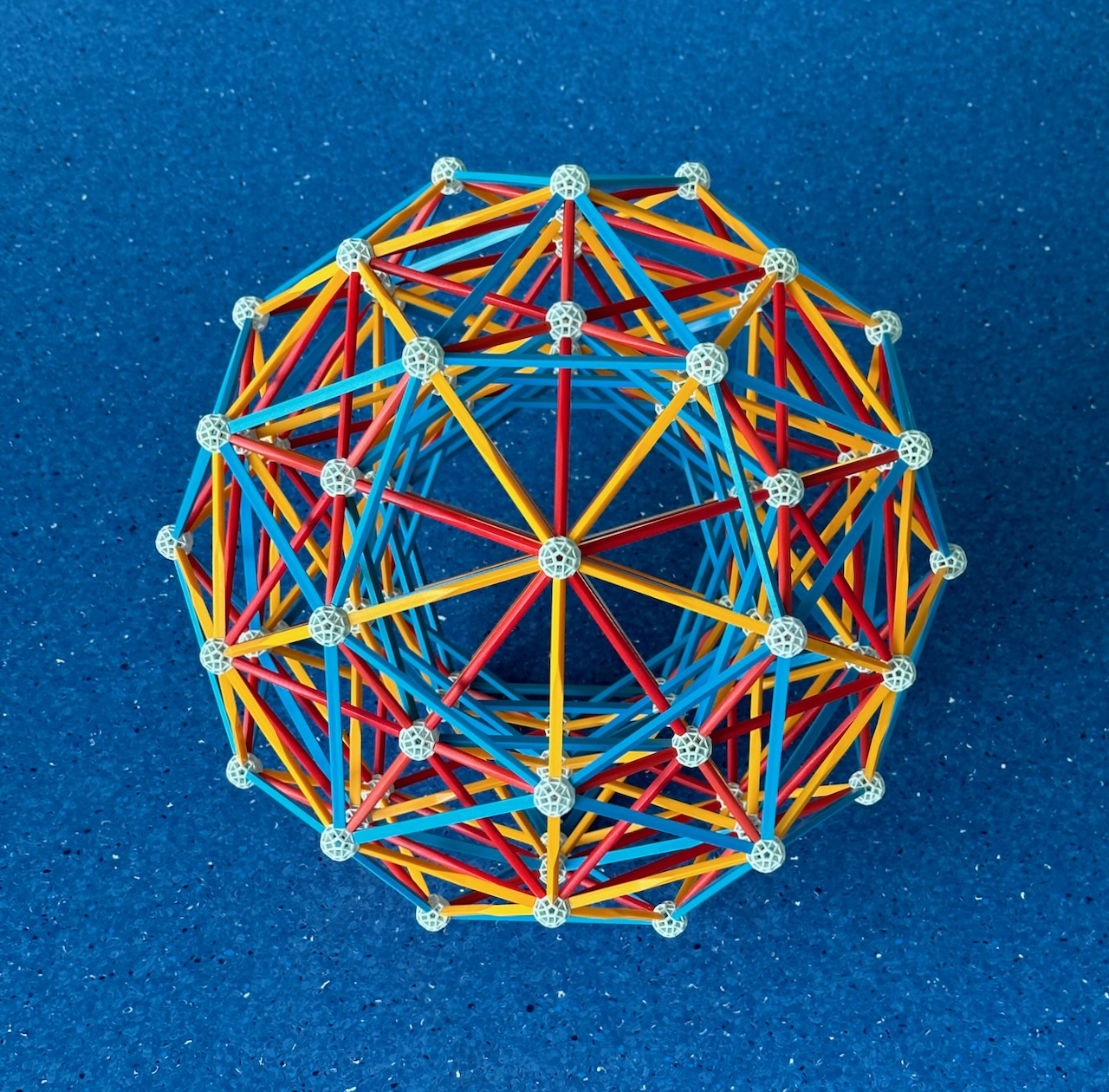Fig. 21: The Icosahedral projection of the 600-cell.

How to build: see instructions in David Richter's list of Zometool projects.

If you followed attentively the construction of the 600-cell, you can check that the 24 pyramidal apices have the same arrangement as the vertices of a 24-cell. Again, the symmetry of the 600-cell implies that there is nothing special about these apices: the 96 vertices of the Snub 24-cell can be decomposed into 4 groups of 24 vertices, each also with the same arrangement as the vertices of a 24-cell. This means that we can build a set of five 24-cells with the same vertex arrangement of the 600-cell. This is a self-dual regular polychoron compound, a model is shown in Figs. 31a and b.

Given the method of construction, the edges of the 600-cell contain those of the Snub 24-cell as a sub-set, i.e., the latter is a diminished 600-cell. Thus, we still see the edges of the central blue Icosahedra of the Snub 24-cell. But apart from that, we can also see see other important sections highlighted by the edges: blue Dodecahedra and Icosidodecahedra. The latter is part of the envelope of the projection; it is therefore an ``Equatorial'' polyhedron (which also has its own equatorial polygon, the Decagon). Studying the model further, you will also see many flattened versions of those polyhedra; these are identical sections located in 3-D planes that are not parallel to ours. This study will make it easier to understand and build some of the models below.

One of these is the dual of the 600-cell; which is necessarily a regular polychoron with the same symmetry of the 600-cell (Hexachosichoric), 600 vertices with Tetrahedral vertex polyhedra, 1200 edges with Triangular figures, 720 Pentagonal faces and 120 Dodecahedral cells: the 120-cell. This is the 4-D equivalent of the Dodecahedron. Its Icosahedral projection appears in Fig. 22. Two of the cells of the 120-cell appear undistorted in the centre of projection: an Icosahedral projection of the 120-cell is necessarily a cell-first projection.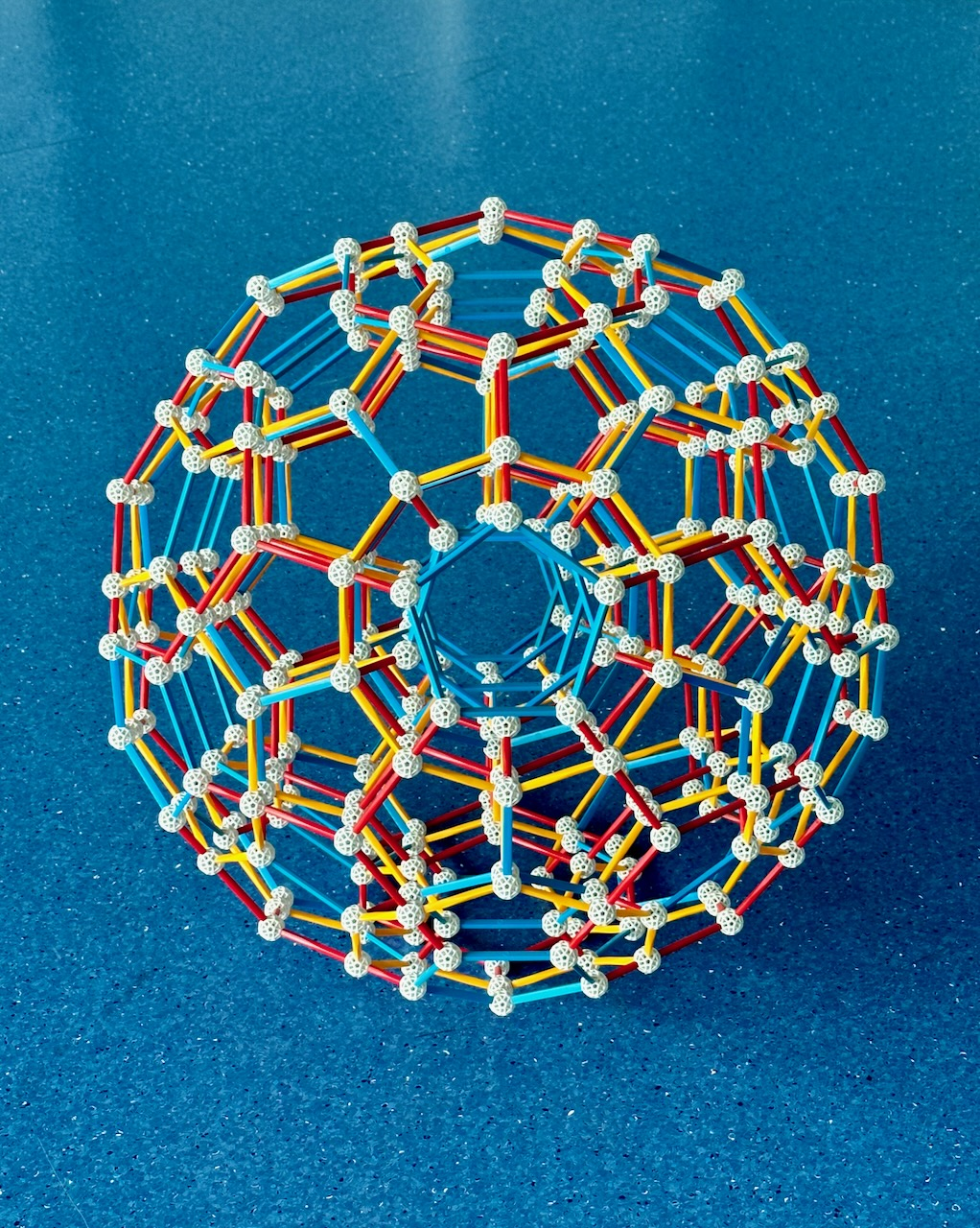Fig. 22: This is the Icosahedral projection of the 120-cell. This projection is the dual of the projection of the 600-cell in Fig. 21.

How to build: see instructions here. The model in the figure is larger than the set sold by Zometool as the hyperdo by φ, the famous golden ratio.

If you pay attention to the model, you will see that all of these projections of Dodecahedral cells can already be seen in the model of the 600-cell above! The reason for this is that, as we will see below, that model also represents the Grand 120-cell, which like the 120-cell, has 120 Dodecahedral cells with the same orientations!

This ends the list of the regular convex polychora.

***

The models above represent only a small number of the 64 convex uniform polychora (the 4-D analogues of the Archimedean polyhedra). Some examples I have not built are in David Richter's list of convex uniform polychora with Pentachoric symmetry and his list of convex uniform polychora with Hexacosichoric symmetry. Many uniform polychora with the symmetries of the 16-cell and 24-cell are not Zomable because they require the construction of Octagons - which are forbidden in the Zometool system.

Among those "Archimedean" polychora there is a third semi-regular polychoron. This results from rectifying the last regular polychoron with Tetrahedral cells, the 600-cell. A model of the Rectified 600-cell is shown in Fig. 23. I have not kept it for lack of Zometool parts, but also because the models of the regular polytopes and their regular compounds already take a lot of space in my office!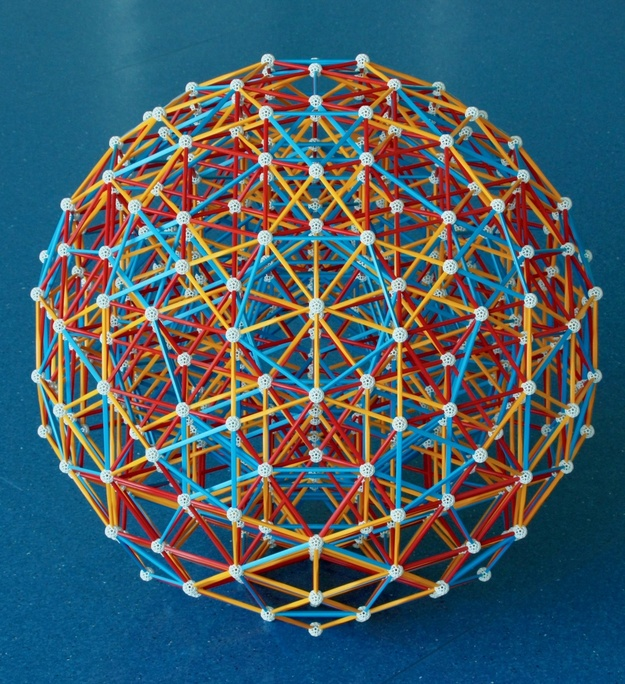Fig. 23: The Icosahedral projection of the Rectified 600-cell. This projection is the rectification of the projection of the 600-cell in Fig. 21.

How to build: After studying the perspective-flattened Icosahedra in the model of the 600-cell, have a look at the Eusebeia page on the Rectified 600 cell. Also recommended is David Richter's page on the rectified 600-cell.

The 600 Tetrahedra of the 600-cell are here replaced by their rectifications, which are Octahedra. In the position of each of the 120 vertices of the 600-cell 120 Icosahedra (the vertex polyhedra of the 600-cell) appeared, two of those appear superposed and without distortion at the very centre of the projection. The vertex polyhedron is the Pentagonal prism. All projections of the Icosahedron that appear in this model are the projections of the Icosahedron we've seen in the model of the 600-cell in Fig. 21.

If you pay close attention to the model, you will be able to see several Archimedean solids in blue, starting with Icosidodecahedra, these are sections of the Rectified 600-cell highlighted by its edges. Studying the model further, you will be able to see many flattened versions of those. Such sections are the cells of 14 non-convex polychora, all of them edge facetings of the Rectified 600-cell and therefore represented by the same model. One of them is the Rectified icosahedral 120-cell, which has Great dodecahedra and Icosidodecahedra as cells, more about the Icosahedral 120-cell below.

These 14 edge-facetings are a sub-group of the 60 facetings of the Rectified 600-cell that are uniform, non-convex polychora.

### Star polychora

We will now present the ten Schläfli-Hess polychora - the regular "star" polychora. These are the 4-D analogues of the regular star polyhedra. You can see their Zometool models rotate, increase in size or even explode with this very cool Observable notebook.

Similarly to what happens in 3 dimensions, all regular star polychora must be stellations and facetings of regular convex polychora because they must also be symmetric around each vertex and around each cell. This means that the cell "planes" and vertices must have the same arrangements as those of one or two regular convex polychora. If the cell plane and vertex arrangements are those of two different convex polychora, then those must be dual to each other because they must have the same overall symmetry. These can only be the 600-cell and / or the 120-cell because, as we will see next, the few regular stellations and facetings of the simpler regular convex polychora are all regular compounds.

Stellating a polychoron extends its cells along their 3-D "planes" until they meet identically extended cells, while faceting remove parts of the polychoron while preserving its vertex arrangement. As for polyhedra (and for the same reasons), if polychora A and B are duals, then the dual of the stellation of A is a faceting of B. It is very important to keep this in mind for understanding what follows. As for polyhedra, the larger the number of cells/vertices of a polychoron, the larger the number of its stellations/facetings.

The different types of stellations and facetings and other geometric transformations between the 120-cell, 600-cell and the regular star polychora are described in detail in Diagrams IIa, b and c below. All these operations preserve the Hexacosichoric symmetry of the 120-cell and 600-cell. Some preliminary conclusions from them:
• In Diagram IIb, we see that nine of the regular star polychora are stellations of the 120-cell, and one of them - the Grand 600-cell - is a stellation of the 600-cell, with 600 Tetrahedra. This latter object is therefore the 4-D analog of the Great Icosahedron, which it has as a vertex polyhedron.
• The dual of each regular star polychoron is also a regular star polychoron; this implies that nine of them are facetings of the 600-cell and that the dual of the Grand 600-cell - the Great grand stellated 120-cell - is a faceting of the 120-cell (see Diagram IIc). This makes it an analog of the Great stellated dodecahedron, which it has as cells.
• In Diagram IIc, we can see that three facetings of the 600-cell - the Icosahedral 120-cell (the cells are the Icosahedral sections visible in the model of the 600-cell in Fig. 21, see virtual model), the Grand 120-cell (the cells are the Dodecahedral sections visible in the model of the 600-cell, see virtual model) and the Great 120-cell (the cells are Great dodecahedra, which have the same edges as the aforementioned Icosahedra, see virtual model) - share its edge arrangement. This means that they are share the Zometool representation of the 600-cell in Fig. 21! This makes them analogues of the Great dodecahedron, which has the edge arrangement of the Icosahedron.
We now present Icosahedral projections of the 7 remaining regular star polychora. Figs. 24a, b, 25b and b represent 6 different facetings of the 600-cell.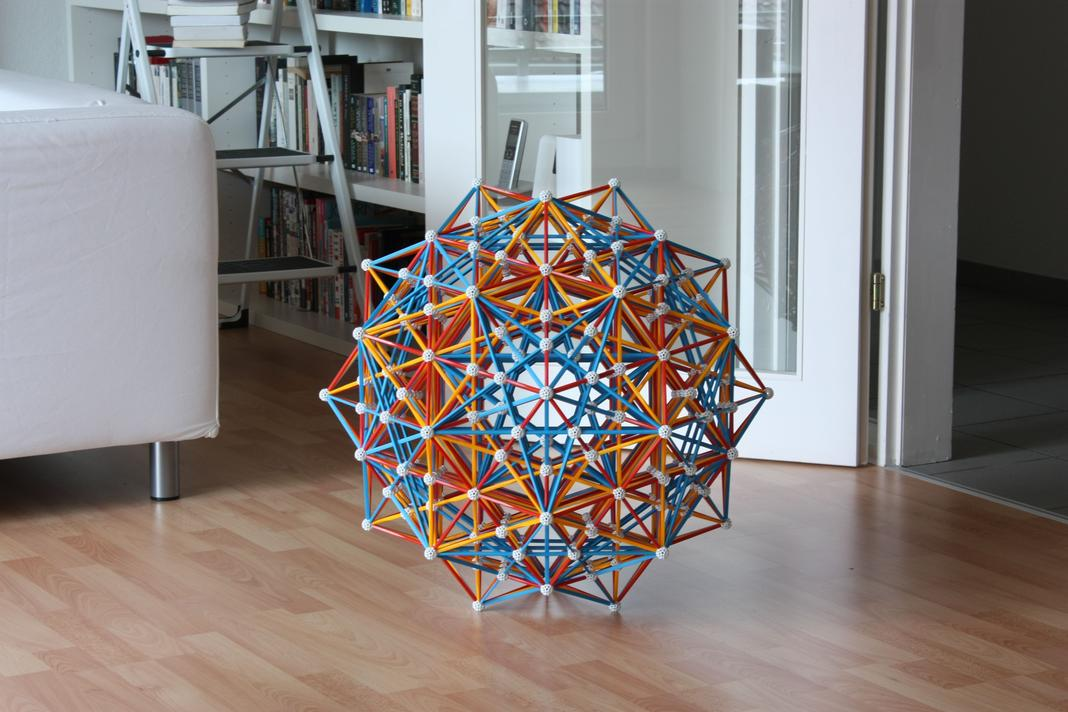Fig. 24a: This model represents the Icosahedral projections of two regular star polychora which share the same edge arrangement, the Small stellated 120-cell (henceforth "Stellated 120-cell" for short, see virtual model) and the Great grand 120-cell (see virtual model).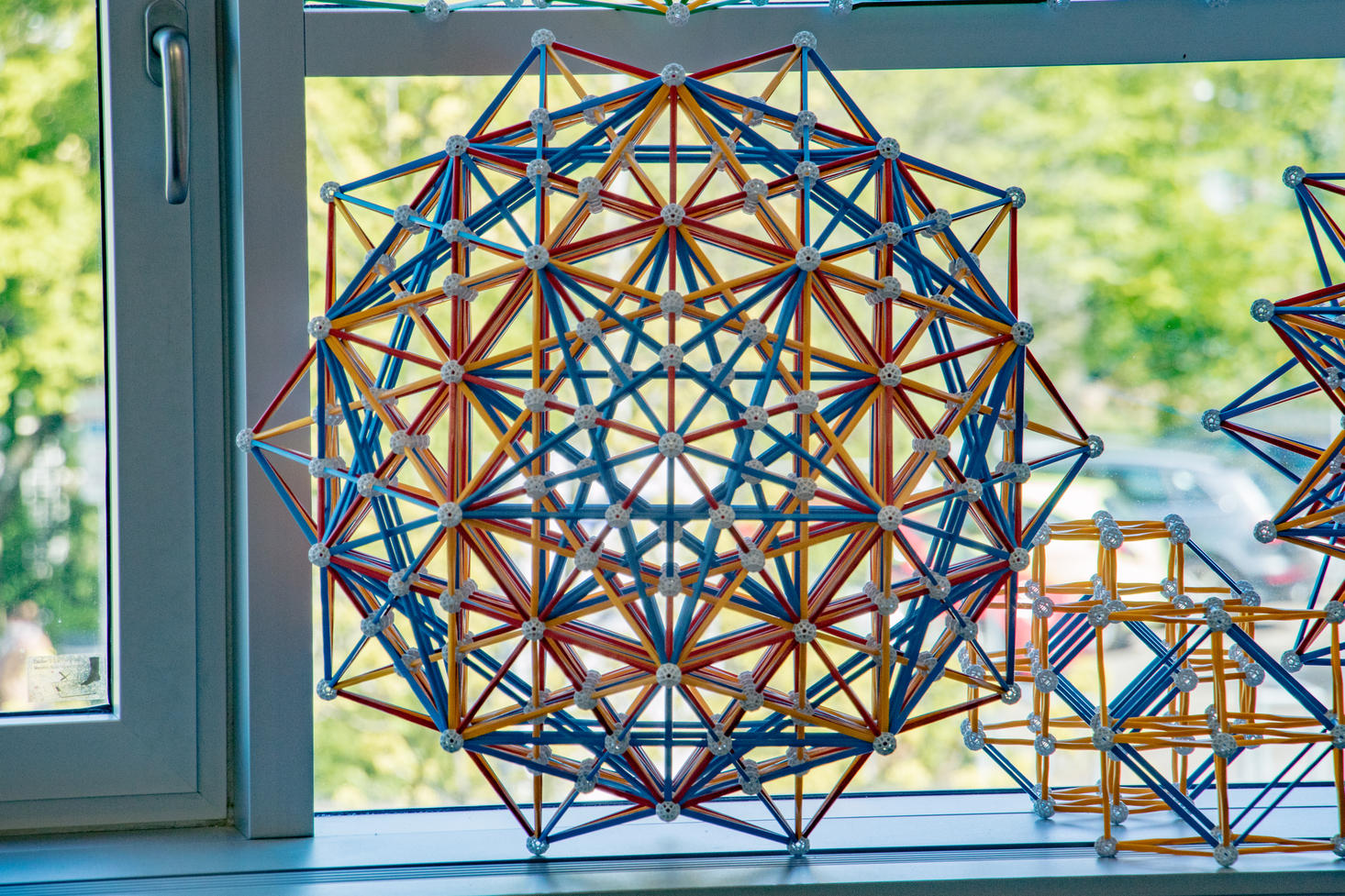Fig. 24b: This is how it looks in my office.
Picture by Jason Wu.

How to build: see instructions in David Richter's dedicated page.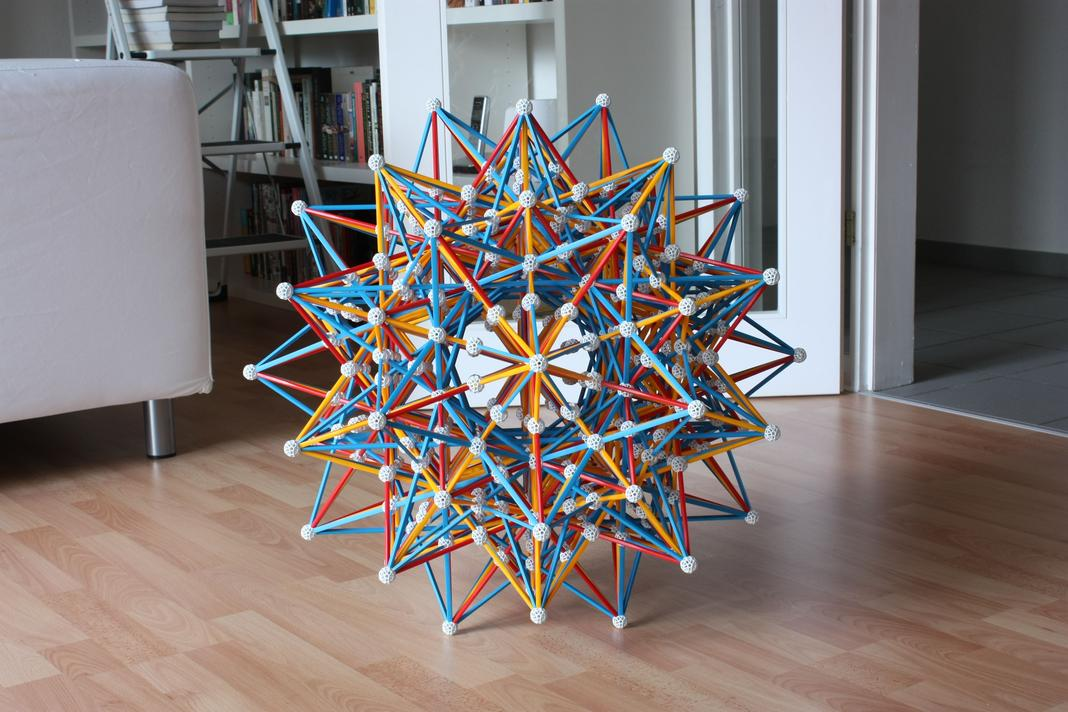Fig. 25a: This model represents the Icosahedral projections of four regular star polychora that share the same edge arrangement: The Grand 600-cell (see virtual model), the Great stellated 120-cell (virtual model), the Grand stellated 120-cell (virtual model) and the Great icosahedral 120-cell (virtual model).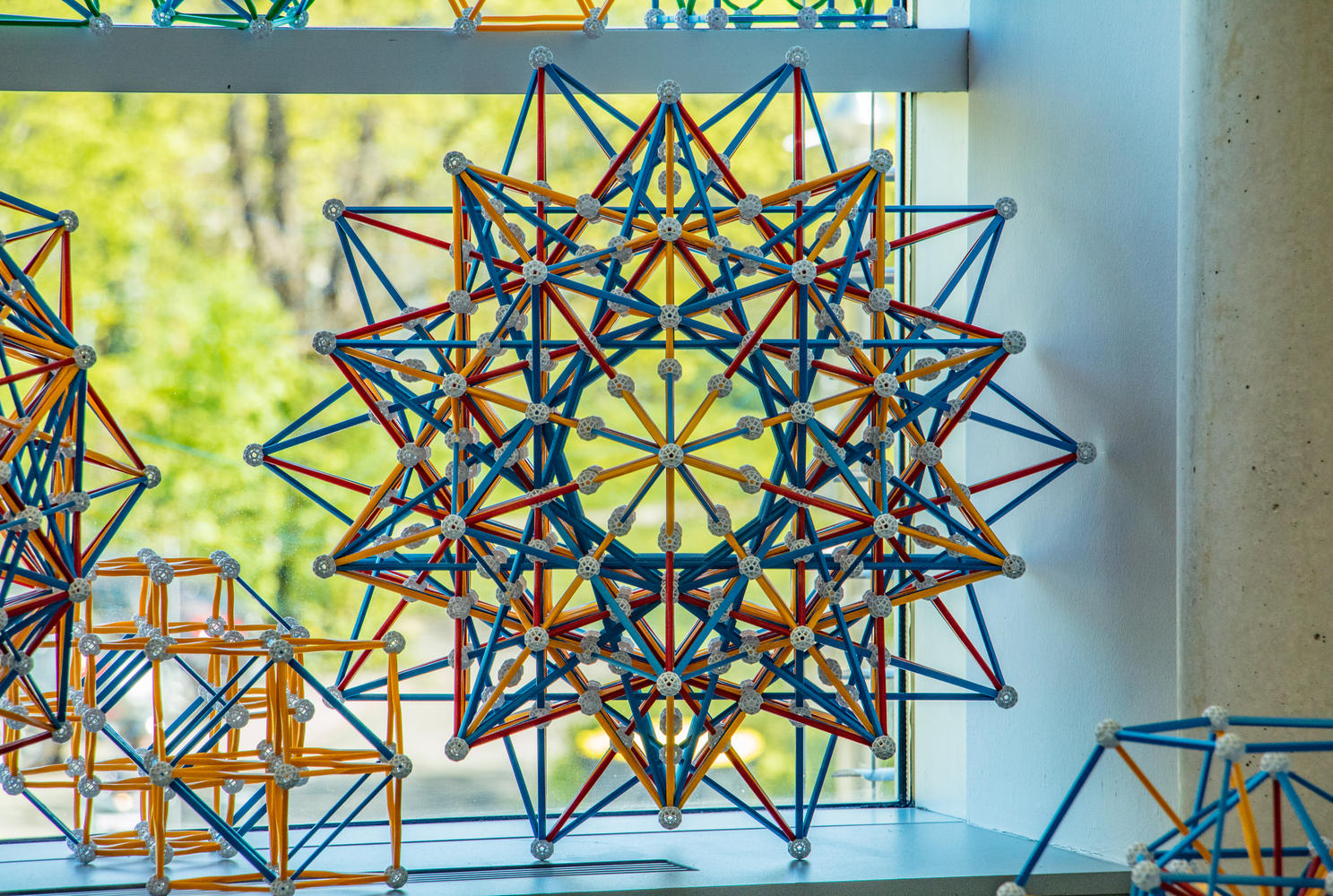Fig. 25b: This is how it looks in my office.
Picture by Jason Wu.

How to build: Make a model of the 600-cell, but extend all internal edges: there can be no broken lines. Then build a new, outer set of vertices of the 600-cell, but increased in size by the Golden ratio, (only a few of these will suffice). For each new outer vertex, see what edges of the internal model point towards it, then extend those edges to the outer vertex. Do this for a set of neighboring vertices, which will allow you to work out the intersections of those edges. Once this is done, apply the same pattern to all outer vertices using Icosahedral symmetry, while removing all the edges of the outer 600-cell that were holding the outer vertices in place. Look here for the justification of this method of construction.
Note that, as in the previous model, there are several intersecting blue edges. Because I wanted to make this a permanent addition to my office, I cut the necessary number of blue struts with pliers.

These Icosahedral projections necessarily have the same vertex arrangement as the Icosahedral projection of the 600-cell (Fig. 21). The difference is that for the model in Figs. 24a and b, the false vertices are those of the Icosahedral projection of the 120-cell (Fig. 22); for the model in Figs. 25a and b they have the same arrangement of a φ smaller 600-cell, at the same scale as the model in Fig. 21.

The models highlight the equatorial polyhedra very well in blue. For the Stellated 120-cell, this is the Dodecadodecahedron, which has the Hexagon as an equatorial polygon. These hexagons are also the equatorial polygons of 24-cells, in a compound of twenty-five of them (see Fig. 33)! For the Grand 600-cell, this is the Great icosidodecahedron, which has a Decagram as an equatorial polygon. As mentioned above, the equatorial polyhedron of the 600-cell is the Icosidodecahedron, therefore the equatorial polyhedra of its facetings must be facetings of the Icosidodecahedron. Still, it is an interesting fact that all aforementioned rectifications of regular polyhedra occur as equatorial polyhedra of regular polychora! All of them have themselves equatorial polygons that are very useful for calculating the metric properties of these polychora.

In Figs. 26a and b, we present the last of the regular star polychora, the most complex of the 16 regular polychora, the final stellation of the 120-cell: The Great grand stellated 120-cell.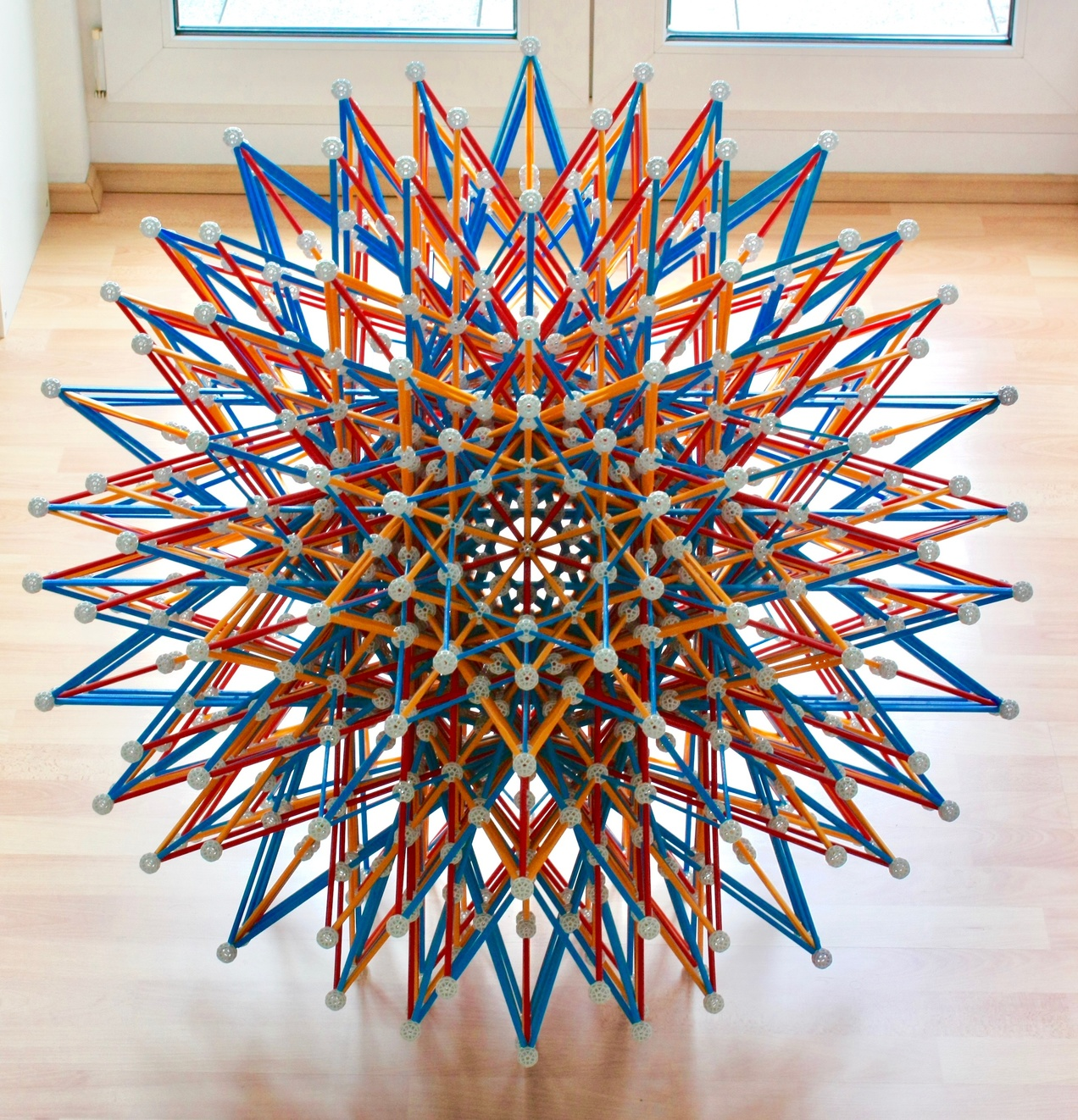Fig. 26a: Icosahedral projection of the Great grand stellated 120-cell, here seen from a 5-fold symmetry axis.Fig. 26b: Same model as in the Figure above, here seen from a 3-fold symmetry axis. For many more perspectives, see a virtual model.

How to build: Make a smaller version of the Stellated 120-cell (in Fig. 24a), but with all internal edges extended - there can be no broken lines. Then identify the equivalent vertices of the largest possible Dodecahedron in the model, i.e., the outermost balls along the 3-fold symmetry axes. These are the vertices of the central Dodecahedron of a (large) 120-cell. Now, based on this, build a few of the remaining "outer" vertices of that 120-cell, at an appropriate scale. Then, from each of those vertices, see what edges in the inner model point towards it, and extend those edges until they reach that vertex. Doing this for a set of neighboring vertices will then allow you to figure out the network of edge intersections. Once this is done, repeat for all vertices of the 120-cell using Icosahedral symmetry, while removing all edges of the large 120-cell that were holding the first set of outer vertices in place. Look here for the justification of this method of construction.
This model can use 20 Y3 struts. These will be directly aligned with the 3-fold symmetry axis shown in this Figure. This saves 40 regular-sized struts (Y2 and Y1) and 20 balls and slightly improves the appearance of the model. This model suffers even more from the blue strut intersections that appear in the previous two models. In this model, I omitted some of those intersections.

With this model, the list of regular polychora is complete!

We now discuss the geometric relations between the 120-cell, 600-cell and the regular star polychora. Instead of 6 polyhedra shown in Diagrams Ia and b, we now have 12 polychora, in Diagrams IIa, b and c we follow Conway, Burgiel and Goodman-Strauss (see references) and arrange them as the vertices of an imaginary Cuboctahedron, which as noticed above has 12 vertices and is the only Archimedean polyhedron that is radially equilateral. This vertex arrangement is, together with the central vertex, part of an optimal sphere packing lattice.

As in those earlier diagrams, the vertical scale indicates the density.
1. Duality. This operation is represented by the two-sided yellow arrows. Since it preserves the density, its arrows are strictly horizontal, as in Diagram Ia. Note that the Great 120-cell and the Grand stellated 120-cell are self-dual.
2. Isomorphism. The red lines that go through the center of the arrangement change a Pentagon into a Pentagram, or vice-versa, in any polygonal element of the polychora at both ends of the line (these elements are either the cell's faces, the cell's vertex figures, or the edge figures). Thus the polychora connected by these red lines are isomorphic; this means that their configuration matrices are identical. An example of this is that the equatorial polyhedra of the 600-cell and Grand 600-cell (Icosidodecahedron and Great icosidodecahedron) are, as remarked in the polyhedron page, isomorphic.

With this Cuboctahedral arrangement, many other geometric relations between these polychora can be represented as sets of parallel lines, as in Diagram Ib. In Diagram IIb we show the four stellation operations that occur among regular polychora; these give them their names.
1. Edge stellation. This operation is represented by the dark blue arrows. It has the same meaning as in 3-D, i.e., the Pentagonal faces are stellated into Pentagrams. This operation preserves the edge figure. As an example, the Stellated 120-cell is formed by stellating each of the 120 Dodecahedra of the 120-cell into a Stellated dodecahedron (Fig. 5). The latter has only 12 vertices (one for each face of the Dodecahedron) arranged as in the Icosahedron. Likewise, the Stellated 120-cell has only 120 vertices - one for each cell of the 120-cell, arranged as in the 600-cell. The vertex figure of the Stellated dodecahedron cells is a Pentagon; thus the vertex polyhedron of the Stellated 120-cell is a regular polyhedron with Pentagonal faces - the Dodecahedron.
2. Greatening. This operation is represented by the light blue arrows; as in 3-D this means replacing a face by a larger dual polygon (see Fig. 11a) in the same 2-D plane. Applying this operation to the 120-cell leads to the Great 120-cell, where each Dodecahedron of the 120-cell is greatened to a Great dodecahedron (Fig. 5). Edge-stellating the Great 120-cell, or greatening the Stellated 120-cell, we obtain the Great stellated 120-cell, where each Dodecahedron of the 120-cell is expanded into a Great stellated dodecahedron. The pattern of cell stellation in the top blue circuit is exactly the one shown in Fig. 11b. Greatening the Icosahedral 120-cell means that the cells of the resulting Great icosahedral 120-cell are the only regular stellation of the Icosahedron, i.e., Great icosahedra.
3. When greatening (above), the faces are replaced by larger dual polygons in the same plane, which are of the same type (see Fig. 11a). When expanding cells within the same 3-D plane we can, apart from the polyhedral transformations of the cells listed above, either expand to larger cells of the same type and orientation or larger dual cells, not both:
1. Aggrandizing. The first operation is depicted by the light green arrows. From this, we see that the cell types in the lower blue circuit are a repeat of the cell types in the top blue circuit we just described. We also see from this that the Grand 600-cell has cells like those of the 600-cell, Tetrahedra.
2. Dual aggrandizing. The second operation is depicted by the dark green arrows. Thus the Icosahedral 120-cell (the dual of the Stellated 120-cell) has 120 Icosahedra as cells.
Even if the cells are self-dual (Tetrahedra), the result of these operations is in principle different, since a Tetrahedron and its dual have different orientations.

In Diagram IIc we show the four faceting operations that occur among regular polychora. These operations can be seen as the duals of the stellation operations in Diagram IIb, i.e., they are like a mirror image of the latter. We now describe them individually.
1. Face faceting. This operation, represented by the solid red and yellow lines, is equivalent to: finding dual + edge stellating + finding dual. Since the edge stellation preserves the edge directions and their edge figures, the dual faceting operation preserves their perpendicular face arrangements; which means that the edge arrangement is also preserved. This operation transforms Pentagonal edge figures into Pentagrams, a consequence of the fact that the dual operation transforms Pentagonal faces into Pentagrams.
2. Edge faceting. This operation, represented by the dashed lines, is equivalent to: finding dual + greatening + finding dual. Since greatening replaces the faces by larger duals in the same planes, the dual faceting operation preserves the edge arrangement (not only the edge directions, but also where they end, since the vertex arrangement is also preserved in any faceting operation) but replaces the edge figures by smaller duals.

These two operations facet the vertex polyhedra, a consequence of the fact that their dual operations stellate the cells. Thus, since the 600-cell has an Icosahedron as a vertex polyhedron, the other 3 polychora in the red circuit have as their vertex polyhedra the 3 facetings of the Icosahedron shown in Fig. 11c: The Icosahedral 120-cell has the Great dodecahedron as vertex polyhedron, the Great 120-cell has the Stellated dodecahedron as vertex polyhedron, and the Grand 120-cell has the Great icosahedron as vertex polyhedron. Since the Stellated 120-cell has the Dodecahedron as vertex polyhedron, its regular faceting, the Great grand 120-cell necessarily has the only regular faceting of the Dodecahedron, the Great stellated dodecahedron, as a vertex polyhedron (see Fig. 12c).

3. The two following faceting operations are the duals of the two aggrandizing operations.
1. Vertex faceting. This operation, represented by the solid blue lines, is equivalent to: finding dual + aggrandizing + finding dual. Since aggrandizing preserves the cell type but enlarges it, the dual faceting operation preserves the type of vertex polyhedron, but reduces its angular size. The light blue lines show that each polychoron in the red family has a faceting in the yellow family with the same type of vertex polyhedron as those we described above. The dark blue line shows that the only faceting of the 120-cell, the Great grand stellated 120-cell, also shares the former's vertex polyhedron, the Tetrahedron.
2. Dual vertex faceting. This operation, represented by the solid brown lines, is equivalent to: finding dual + dual aggrandizing + finding dual. Since dual aggrandizing preserves the cell plane but replaces the cells by larger dual cells in the same 3-D plane, the dual faceting operation transforms the vertex polyhedron into its dual, while reducing its angular size.
Importance of Diagram IIc for Zometool models: Since Zometool models represent vertex and edge arrangements, the first two operations preserve the Zometool representations! Indeed, the 4 polychora in the red circuit are represented by the model of the 600-cell (Fig. 21) and the 4 polychora in the yellow circuit are represented by the model of the Grand 600-cell (Figs. 25a and b). The 2 polychora in the dashed brown line are represented by the model of the Stellated 120-cell (Figs. 24a and b). The Zometool models of the 120-cell (Fig. 22) and the Great grand stellated 120-cell (Figs 26a and b) represent only those polychora.

In the Figure below, we show a Zometool representation of Diagrams IIa, b and c.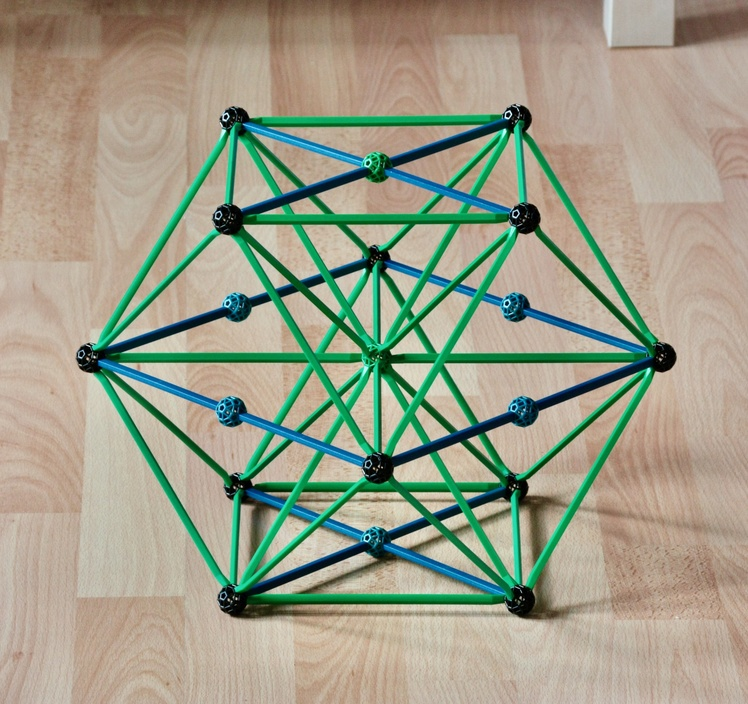Fig. 27: Zometool representation of diagrams IIa, b and c.

The black balls - the vertices of the outer Cuboctahedron - represent the 120-cell, 600-cell and the 10 regular star polychora, the struts represent the geometrical transformations discussed above. Note how the edge stellation and its dual, the face faceting, are special, being the only transformations represented by the blue struts. All others, including duality, can be represented by green struts and include special instances going through the centre of the figure, the isomorphisms.

Challenge to the reader: As mentioned above, the Cuboctahedron has Hexagons as "equatorial" polygons. How many? In Diagrams IIa, b and c, which equatorial Hexagon touches polychora where the cells match the polyhedra touched by the Hexagon in Diagrams Ia and b? Do the geometric transformations along that Hexagon match those in Diagrams Ia and b?
Clue: There is a type of stellation that exists only in that Hexagon, but it does not correspond to a stellation in Diagrams Ia and b, but to another transformation. Which one?

***

Currently we know of 2188 uniform polychora. It is not known if the list is complete or not, but it likely isn't, because hundreds of them have been found since 2020. Clearly, the vast majority of these are neither regular nor convex: they are the uniform ``star'' polychora.

Some of these are edge facetings of polychora described above, they are therefore represented by the same Zometool models. As an example, the aforementioned edge facetings of the Rectified 600-cell are all represented by the model of the Rectified 600-cell in Fig. 23.

The only faceting of the 16-cell, the Tesseractihemioctachoron, is an edge faceting; so it shares the Zometool representations of the 16-cell. This polychoron is constructed with the 4 equatorial Octahedra of the 16 cell plus 8 of its 16 Tetrahedra. Since all cells are regular, this is a semi-regular non-convex polychoron. It is the 4-dimensional analog of the Tetrahemihexahedron, which it has as vertex polyhedron; which is necessarily a faceting of the Octahedral vertex figure of the 16-cell.

The Dodecahedral vertex polyhedron of the Stellated 120-cell (which, as we saw in Diagram IIc, is unique among all regular polychora) can be faceted in dozens of ways, yielding dozens of uniform edge facetings of the Stellated 120-cell. In Diagram IIc, we used its only regular faceting, the Great stellated dodecahedron, to obtain the only regular faceting of the Stellated 120-cell, the Great grand 120-cell. If we use instead the ditrigonal polyhedra in Fig. 7, we obtain three additional semi-regular polychora:
1. With the Small ditrigonal icosidodecahedron we obtain the Small ditrigonal 120-600-cell, which has 120 Great icosahedra and 600 Tetrahedra as cells (this name is consistent with those of the quasi-regular polyhedra, which indicate the number of the two types of faces separately). These Great icosahedra have the same edges as the Stellated dodecahedra of the Stellated 120-cell.
2. With the Ditrigonal dodecadodecahedron we obtain the Ditrigonal 120-120-cell, which has 120 Great icosahedra and 120 Icosahedra as cells. These Icosahedra can be seen when we build the model.
3. Finally, with the Great ditrigonal icosidodecahedron we obtain the Great ditrigonal 120-600-cell, which has 120 Icosahedra and 600 Tetrahedra as cells.
In addition to sharing the vertex and edge arrangement of the Stellated 120-cell (and thus sharing the same Zometool representation), these three polychora share among themselves an identical face arrangement, a consequence of the fact that their vertex polyhedra, the ditrigonal polyhedra, share the same edge arrangement.

Being edge facetings of regular polychora, the Tesseractihemioctachoron and the three ditrigonal polychora are isogonal and isotoxal. Furthermore, their vertex polyhedra are also isotoxal, which each edge separating the two types of faces; this implies that these four polychora are also isohedral, whith each face (Triangles in all four cases) separating the two kinds of cells, all of which are regular. No other semi-regular polychora come this close to full regularity.

Additionally, two of the facetings of this Dodecahedral vertex polyhedron are regular compounds: the Compound of five cubes and the Compound of ten tetrahedra. This is important: as we will see later, this implies that some regular polychoron compounds are Zomable.

Main polytope page.